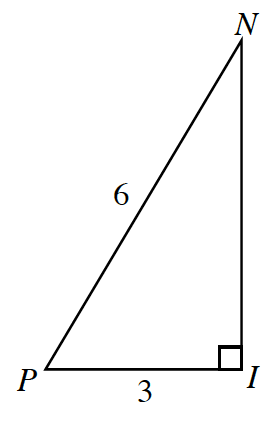### Home > PC3 > Chapter 1 > Lesson 1.1.3 > Problem1-25

1-25.Use $ΔPIN$ to complete the parts below.

1. Calculate the exact length of $IN$.

Use the Pythagorean Theorem.

2. Write and simplify a ratio for $\cos(P)$.

$\cos(P) = \frac{\text{adjacent}}{\text{hypotenuse}}$

3. Write and simplify a ratio for $\sin(P)$.

$\sin(P) = \frac{\text{opposite}}{\text{hypotenuse}}$